### Jackknife Method

The jackknife method of variance estimation deletes one PSU at a time from the full sample to create replicates. This method is also known as the delete-1 jackknife method because it deletes exactly one PSU in every replicate. The total number of replicatesis the same as the total number of PSUs. In each replicate, the sampling weights of the remaining PSUs are modified by the jackknife coefficient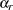. The modified weights are called replicate weights.

Let PSUin stratum
be omitted for theth replicate; then the jackknife coefficient and replicate weights are computed as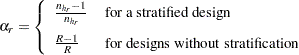and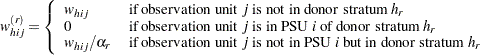You can use the VARMETHOD=JACKKNIFE(OUTJKCOEFS=) method-option to store the jackknife coefficients in a SAS data set and use the VARMETHOD=JACKKNIFE(OUTWEIGHTS=) method-option to store the replicate weights in a SAS data set.

If you provide your own replicate weights with a REPWEIGHTS statement, then you can also provide corresponding jackknife coefficients with the JKCOEFS= option. If you provide replicate weights with a REPWEIGHTS statement but do not provide jackknife coefficients, then the procedure uses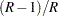as the default jackknife coefficient for every replicate, whereis the total number of replicates.

Let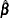be the estimated proportional hazards regression coefficients from the full sample, and let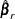be the estimated regression coefficients for theth replicate. PROC SURVEYPHREG estimates the covariance matrix ofby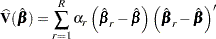with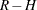degrees of freedom, whereis the number of replicates and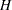is the number of strata, or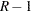when there is no stratification.

If one or more components ofcannot be calculated for some replicates, then the variance estimator uses only the replicates for which the proportional hazards regression coefficients can be estimated. Estimability and nonconvergence are two common reasons whymight not be available for a replicate sample even ifis defined for the full sample. Letbe the number of replicates whereare available and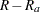be the number of replicates whereare not available. Without loss of generality, assume that the firstreplicates are available; then the jackknife variance estimator is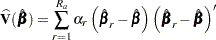with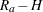degrees of freedom, whereis the number of strata. Alternatively, you can use the VADJUST=AVGREPSS option in the MODEL statement to use the average sum of squares for the invalid replicate samples. See Variance Adjustment Factors for details.